# Square2

Side of the square is a = 6.2 cm, how long is its diagonal?

Result

d =  8.77 cm

#### Solution:

$d^2 = a^2 + a^2 \ \\ d = \sqrt2 a = \sqrt2 \cdot 6.2 = 8.77 \ \text { cm }$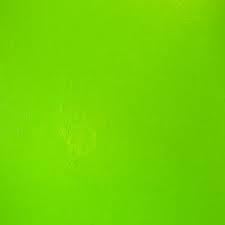Our examples were largely sent or created by pupils and students themselves. Therefore, we would be pleased if you could send us any errors you found, spelling mistakes, or rephasing the example. Thank you!

Leave us a comment of this math problem and its solution (i.e. if it is still somewhat unclear...):Be the first to comment!#### Following knowledge from mathematics are needed to solve this word math problem:

Pythagorean theorem is the base for the right triangle calculator.

## Next similar math problems:

1. Dog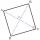Dog is tied to a chain, which is mounted in a corner of the yard. Yard has the shape of a square with a side length of 20 meters. The same long is also dogchain. Are there places in the yard where dog can't reach?
2. Tree trunkFrom the tree trunk, the diameter at the narrower end is 28 cm, a beam of square cross-section is to be made. Calculate the longest side of the largest possible square cross-section.
3. Square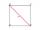Calculate the side of a square with a diagonal measurement 10 cm.
4. Two diagonalsThe rhombus has a side length 12 cm and length of one diagonal 21 cm. What is the length of the second diagonal?
5. Square side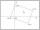Calculate length of side square ABCD with vertex A[0, 0] if diagonal BD lies on line p: -4x -5 =0.
6. InfinityIn a square with side 18 is inscribed circle, in circle is inscribed next square, again circle and so on to infinity. Calculate the sum of area of all these squares.
7. Right angled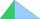From the right triangle with legs 12 cm and 20 cm we built a square with the same content as the triangle. How long will be side of the square?
8. Square diagonalCalculate the length of diagonal of the square with side a = 23 cm.
9. SquareRectangular square has side lengths 183 and 244 meters. How many meters will measure the path that leads straight diagonally from one corner to the other?
10. OctagonWe have a square with side 84 cm. We cut the corners to make his octagon. What will be the side of the octagon?
11. EstateEstate shaped rectangular trapezoid has bases long 34 m , 63 m and perpendicular arm 37 m. Calculate how long is its fence.
12. The cellar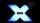Mr Novák has a cellar and a cellar window in the chalet has 0.6 meter square window. The window wishes to place an X-shaped grid in a square. He uses iron welded bars. Calculate the lengths of individual bars and what the total length of the bars he has t
13. SquareCalculate area of the square with diagonal 64 cm.
14. Median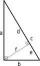In the right triangle are sides a=41 dm b=42 dm. Calculate the length of the medians tc to the hypotenuse.
15. The ditchDitch with cross section of an isosceles trapezoid with bases 2m 6m are deep 1.5m. How long is the slope of the ditch?
16. SatinSanusha buys a piece of satin 2.4 m wide. The diagonal length of the fabric is 4m. What is the length of the piece of satin?
17. HeightIs right that in any right triangle height is less or equal half of the hypotenuse?